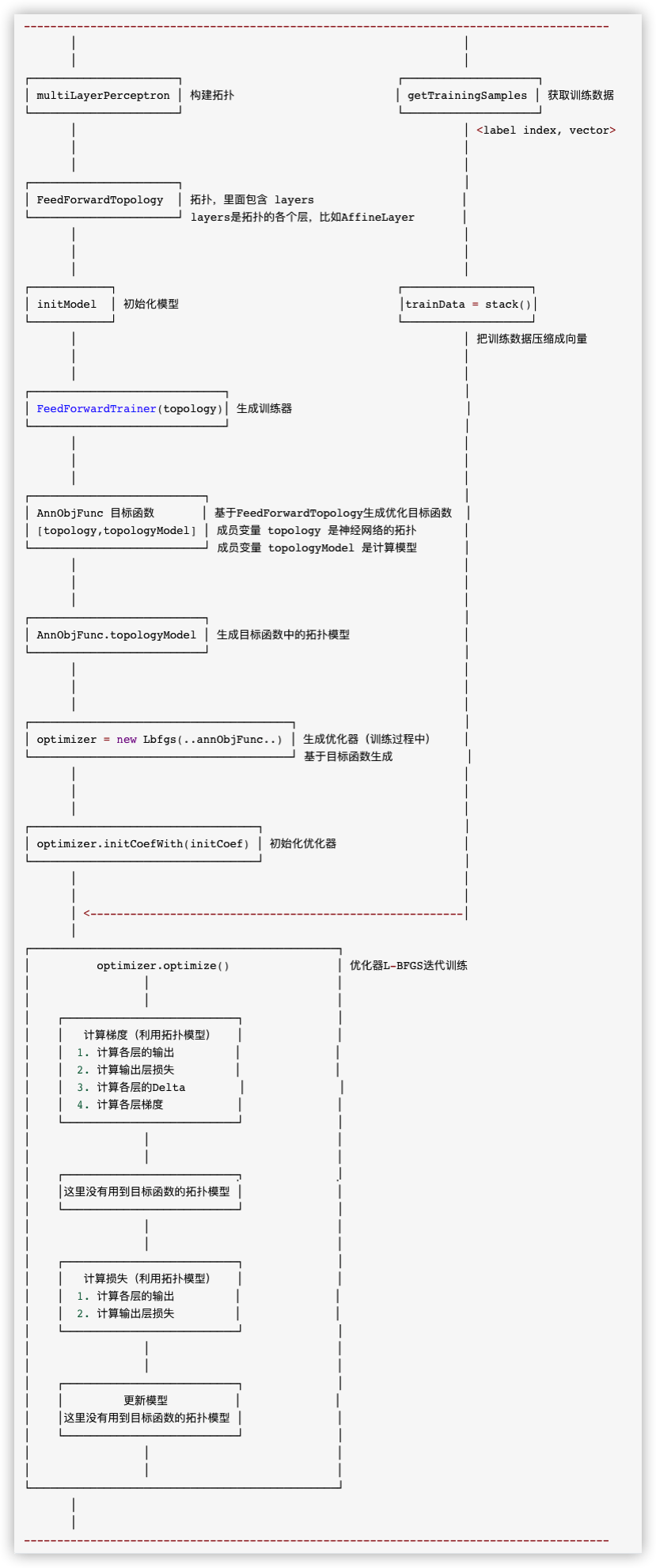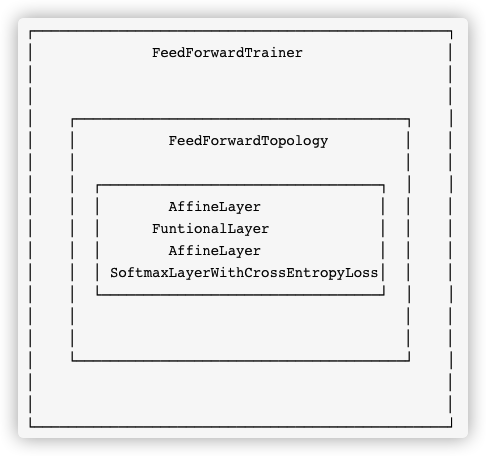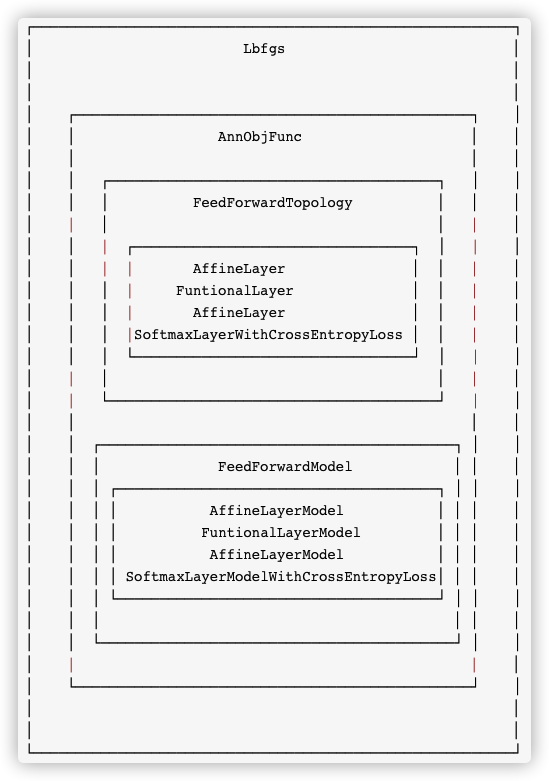## 0x01 背景概念

### 1.2 反向传播

• 代价信息如何传递到深度模型的每一层？
• 基于传递到本层的信息，本层的参数应该如何更新？

### 1.3 代价函数

• 代价函数必须能够表达为平均值。

• 代价函数不能依赖于输出层旁边网络的任何激活值。

### 1.4 优化过程

#### 1.4.2 梯度下降

$x_{n+1} = x_n – η\frac{df(x)}{dx}$

### 1.5 相关公式

#### 1.5.1 加权求和 h

$h_j = \sum_{i=0}^M w_{ij}x_j$

hj 表示当前节点的所有输入加权之和。

#### 1.5.2 神经元输出值 a

$a_j = g(h_j) = g(\sum_{i=0}^M w_{ij}x_j)$

• a_j 表示隐藏层神经元的输出值。
• g()代表激活函数，w是权重，x是输入。
• a_j=x_jk 即当前层神经元的输出值，等于下一层神经元的输入值。

#### 1.5.3 输出层的输出值 y

$y = a_k = g(h_k) = g(\sum_{i=0}^M w_{jk}x_{jk})$

• y 表示输出层的值，也就是最终结果。
• h_k 表示输出层神经元k的输入加权之和。

#### 1.5.4 激活函数g(h)

$g(h)=σ(h)= \frac{1}{1+e^{-h}}$

sigmoid函数的导数：

$σ ′(x)=σ(x)[1−σ(x)]$

$g ′ (h)=a_j (1−a_j )$

#### 1.5.5 损失函数E

$E = \frac{1}{2}\sum _{k=1}^N(y-t)^2$

• 平方是为了避免超平面两端的误差点相互抵消（y−t 存在正负）。
• 前面系数取1/2 是为了之后采用梯度下降时，求梯度（偏导数）时能抵消平方求导后的2。

#### 1.5.6 误差反向传播——更新权重

$\frac{∂E}{∂w_{ik}} = \frac{∂E}{∂h_k} \frac{∂h_k}{∂w_{ik}}$

h_k表示输出层神经元k的所有输入加权之和，也就是激活函数g(h)的输入值。

#### 1.5.7 输出层增量项 δo

$δ_o(k) = \frac{∂E}{∂h_{k}} = \frac{∂E}{∂y} \frac{∂y}{∂h_k} = (y−t)g ′(h_ k )$

#### 1.5.8 更新输出层权重wjk

$w_{jk} ←w _{jk} −η \frac{∂E}{∂w_{jk}}$

$w_{jk} = w_{jk} – ηδ_o(k)a_i$

ai是上一层的输出值，也即是输出层的输入值xi。

## 0x02 示例代码

public class MultilayerPerceptronClassifierExample {
public static void main(String[] args) throws Exception {
BatchOperator data = Iris.getBatchData();

MultilayerPerceptronClassifier classifier = new MultilayerPerceptronClassifier()
.setFeatureCols(Iris.getFeatureColNames())
.setLabelCol(Iris.getLabelColName())
.setLayers(new int[]{4, 5, 3})
.setMaxIter(100)
.setPredictionCol("pred_label")
.setPredictionDetailCol("pred_detail");

BatchOperator res = classifier.fit(data).transform(data);

res.print();
}
}


Iris定义如下

public class Iris {
final static String URL = "https://alink-release.oss-cn-beijing.aliyuncs.com/data-files/iris.csv";
final static String SCHEMA_STR
= "sepal_length double, sepal_width double, petal_length double, petal_width double, category string";

public static BatchOperator getBatchData() {
return new CsvSourceBatchOp(URL, SCHEMA_STR);
}

public static StreamOperator getStreamData() {
return new CsvSourceStreamOp(URL, SCHEMA_STR);
}

public static String getLabelColName() {
return "category";
}

public static String[] getFeatureColNames() {
return new String[] {"sepal_length", "sepal_width", "petal_length", "petal_width"};
}
}


## 0x03 训练总体逻辑

MultilayerPerceptronTrainBatchOp 类是批处理训练的实现。

protected BatchOperator train(BatchOperator in) {
}


• 1）获取一些元信息，比如label名称，特征列名，特征类型等；
• 2）获取测试数据 trainData = getTrainingSamples
• 3）训练
• 3.1）获取初始权重 initialWeights = getInitialWeights();
• 3.2）构建拓扑 topology = FeedForwardTopology.multiLayerPerceptron
• 3.3）构建训练器 FeedForwardTrainer
• 3.3.1）初始化模型
• 3.3.2）构建目标函数
• 3.3.3）训练器会基于目标函数构建优化器，这里的优化器是L-BFGS
• 3.4）训练获取最终权重 weights = trainer.train
• 4）输出模型 DataSet<Row>；
• 5）把DataSet<Row>转成Table；
@Override
BatchOperator<?> in = checkAndGetFirst(inputs);

// 1）获取一些元信息，比如label名称，特征列名，特征类型等。
final String labelColName = getLabelCol();
final String vectorColName = getVectorCol();
final boolean isVectorInput = !StringUtils.isNullOrWhitespaceOnly(vectorColName);
final String[] featureColNames = isVectorInput ? null :
(getParams().contains(FEATURE_COLS) ? getFeatureCols() :
TableUtil.getNumericCols(in.getSchema(), new String[]{labelColName}));

final TypeInformation<?> labelType = in.getColTypes()[TableUtil.findColIndex(in.getColNames(),
labelColName)];
DataSet<Tuple2<Long, Object>> labels = getDistinctLabels(in, labelColName);

// 此处程序变量如下：
labelColName = "category"
vectorColName = null
isVectorInput = false
featureColNames = {String@6412}
0 = "sepal_length"
1 = "sepal_width"
2 = "petal_length"
3 = "petal_width"
labelType = {BasicTypeInfo@6414} "String"
labels = {MapOperator@6415}

// 2）获取测试数据
// get train data
DataSet<Tuple2<Double, DenseVector>> trainData =
getTrainingSamples(in, labels, featureColNames, vectorColName, labelColName);

// train 3）训练
final int[] layerSize = getLayers();
final int blockSize = getBlockSize();
// 3.1）获取初始权重
final DenseVector initialWeights = getInitialWeights();
// 3.2）获取拓扑
Topology topology = FeedForwardTopology.multiLayerPerceptron(layerSize, true);
// 3.3）构建训练器
FeedForwardTrainer trainer = new FeedForwardTrainer(topology,
layerSize, layerSize[layerSize.length - 1], true, blockSize, initialWeights);
// 3.4）训练获取最终权重
DataSet<DenseVector> weights = trainer.train(trainData, getParams());

// output model 4）输出模型
DataSet<Row> modelRows = weights
.flatMap(new RichFlatMapFunction<DenseVector, Row>() {
@Override
public void flatMap(DenseVector value, Collector<Row> out) throws Exception {
Object[] labels = new Object[bcLabels.size()];
bcLabels.forEach(t2 -> {
labels[t2.f0.intValue()] = t2.f1;
});

MlpcModelData model = new MlpcModelData(labelType);
model.labels = Arrays.asList(labels);
model.meta.set(ModelParamName.IS_VECTOR_INPUT, isVectorInput);
model.meta.set(MultilayerPerceptronTrainParams.LAYERS, layerSize);
model.meta.set(MultilayerPerceptronTrainParams.VECTOR_COL, vectorColName);
model.meta.set(MultilayerPerceptronTrainParams.FEATURE_COLS, featureColNames);
model.weights = value;
new MlpcModelDataConverter(labelType).save(model, out);
}
})

// 5）把DataSet<Row>转成Table
setOutput(modelRows, new MlpcModelDataConverter(labelType).getModelSchema());
}


### 3.1 总体逻辑示例图

----------------------------------------------------------------------------------------
│                                                          │
│                                                          │
┌──────────────────────┐                    		┌────────────────────┐
│ multiLayerPerceptron │ 构建拓扑                        │ getTrainingSamples │ 获取训练数据
└──────────────────────┘                                └────────────────────┘
│                                                          │ <label index, vector>
│                                                          │
│                                                          │
┌──────────────────────┐                                          │
│ FeedForwardTopology  │ 拓扑，里面包含 layers                      │
└──────────────────────┘ layers是拓扑的各个层，比如AffineLayer       │
│                                                          │
│                                                          │
│                                                          │
┌────────────┐                    		        ┌────────────────────┐
│ initModel  │ 初始化模型                                │trainData = stack() │
└────────────┘                                          └────────────────────┘
│                                                          │ 把训练数据压缩成向量
│                                                          │
│                                                          │
┌─────────────────────────────┐                                   │
│ FeedForwardTrainer(topology)│ 生成训练器                          │
└─────────────────────────────┘                                   │
│                                                          │
│                                                          │
│                                                          │
┌──────────────────────────┐                                      │
│ AnnObjFunc 目标函数       │ 基于FeedForwardTopology生成优化目标函数  │
│ [topology,topologyModel] │ 成员变量 topology 是神经网络的拓扑       │
└──────────────────────────┘ 成员变量 topologyModel 是计算模型       │
│                                                          │
│                                                          │
│                                                          │
┌──────────────────────────┐                                      │
│ AnnObjFunc.topologyModel │ 生成目标函数中的拓扑模型                 │
└──────────────────────────┘                                      │
│                                                          │
│                                                          │
│                                                          │
┌───────────────────────────────────────┐                         │
│ optimizer = new Lbfgs(..annObjFunc..) │ 生成优化器（训练过程中）    │
└───────────────────────────────────────┘ 基于目标函数生成           │
│                                                          │
│                                                          │
│                                                          │
┌──────────────────────────────────┐                              │
│ optimizer.initCoefWith(initCoef) │ 初始化优化器                   │
└──────────────────────────────────┘                              │
│                                                          │
│                                                          │
│ <--------------------------------------------------------│
│
┌──────────────────────────────────────────────┐
│          optimizer.optimize()                │ 优化器L-BFGS迭代训练
│                 │                            │
│                 │                            │
│    ┌──────────────────────────┐              │
│    │   计算梯度（利用拓扑模型）   │              │
│    │  1. 计算各层的输出         │              │
│    │  2. 计算输出层损失         │              │
│    │  3. 计算各层的Delta        │              │
│    │  4. 计算各层梯度           │              │
│    └──────────────────────────┘              │
│                 │                            │
│                 │                            │
│    ┌──────────────────────────┐              │
│    │         计算方向          │              │
│    │这里没有用到目标函数的拓扑模型 │              │
│    └──────────────────────────┘              │
│                 │                            │
│                 │                            │
│    ┌──────────────────────────┐              │
│    │   计算损失（利用拓扑模型）   │              │
│    │  1. 计算各层的输出         │              │
│    │  2. 计算输出层损失         │              │
│    └──────────────────────────┘              │
│                 │                            │
│                 │                            │
│    ┌──────────────────────────┐              │
│    │         更新模型          │              │
│    │这里没有用到目标函数的拓扑模型 │              │
│    └──────────────────────────┘              │
│                 │                            │
│                 │                            │
└──────────────────────────────────────────────┘
│
│
----------------------------------------------------------------------------------------Django序列化组件Serializers详解

### 3.2 L-BFGS训练调用逻辑概述

• CalcGradient() 计算梯度
• CalDirection(...) 计算方向
• CalcLosses(...) 计算损失
• UpdateModel(...) 更新模型

• 1）调用 AnnObjFunc.updateGradient；
• 1.1）调用 目标函数中拓扑模型 topologyModel.computeGradient 来计算
• 1.1.1）计算各层的输出；forward(data, true)
• 1.1.2）计算输出层损失；labelWithError.loss
• 1.1.3）计算各层的Delta；layerModels.get(i).computePrevDelta
• 1.1.4）计算各层梯度；layerModels.get(i).grad

CalDirection 计算方向

• 这里没有用到目标函数的拓扑模型。

CalcLosses 计算损失

• 1）调用 AnnObjFunc.calcSearchValues; 其内部会调用 calcLoss 计算损失；
• 1.1）调用 topologyModel.computeGradient 来计算损失
• 1.1.1）计算各层的输出；forward(data, true)
• 1.1.2）计算输出层损失；labelWithError.loss

UpdateModel 更新模型

• 这里没有用到目标函数的拓扑模型。

### 3.3 获取训练数据

getTrainingSamples函数将从原始输入获取训练数据。

5.1	3.5	1.4	0.2	Iris-setosa
5	2	3.5	1	Iris-versicolor
5.1	3.7	1.5	0.4	Iris-setosa
6.4	2.8	5.6	2.2	Iris-virginica
6	2.9	4.5	1.5	Iris-versicolor


• 1）获取元数据，比如特征列的index，label列的index；
• 2）把labels广播，后续会在open函数中使用；
• 3）open函数中得倒一个 label : index 的映射
• 4）map 函数中有两种执行序列，都会转换为 <label index, vector> 这样的二元组
• 4.1）原始输入中有vector，比如类似 5.1 3.5 1.4 0.2 Iris-setosa 5.1 3.5 1.4 0.2，这些加粗的就是vector。
• 4.2）原始输入中没有vector，比如类似 5.1 3.5 1.4 0.2 Iris-setosa ；

private static DataSet<Tuple2<Double, DenseVector>> getTrainingSamples(
BatchOperator data, DataSet<Tuple2<Long, Object>> labels,
final String[] featureColNames, final String vectorColName, final String labelColName) {

// 1）获取元数据，比如特征列的index，label列的index；
final boolean isVectorInput = !StringUtils.isNullOrWhitespaceOnly(vectorColName);
final int vectorColIdx = isVectorInput ? TableUtil.findColIndex(data.getColNames(), vectorColName) : -1;
final int[] featureColIdx = isVectorInput ? null : TableUtil.findColIndices(data.getSchema(),
featureColNames);
final int labelColIdx = TableUtil.findColIndex(data.getColNames(), labelColName);

// 程序变量如下
isVectorInput = false
vectorColIdx = -1
featureColIdx = {int@6443}
0 = 0
1 = 1
2 = 2
3 = 3
labelColIdx = 4

DataSet<Row> dataRows = data.getDataSet();
return dataRows
.map(new RichMapFunction<Row, Tuple2<Double, DenseVector>>() {
transient Map<Comparable, Long> label2index;

@Override
public void open(Configuration parameters) throws Exception {
this.label2index = new HashMap<>();
// 得倒一个label : index 的映射
bcLabels.forEach(t2 -> {
Long index = t2.f0;
Comparable label = (Comparable) t2.f1;
this.label2index.put(label, index);
});
// 变量是
this = {MultilayerPerceptronTrainBatchOp\$2@11578}
label2index = {HashMap@11580}  size = 3
"Iris-versicolor" -> {Long@11590} 2
"Iris-virginica" -> {Long@11592} 1
"Iris-setosa" -> {Long@11594} 0

}

@Override
public Tuple2<Double, DenseVector> map(Row value) throws Exception {
Comparable label = (Comparable) value.getField(labelColIdx);
Long labelIdx = this.label2index.get(label);

if (isVectorInput) { // 4.1）如果原始输入中有vector
Vector vec = VectorUtil.getVector(value.getField(vectorColIdx));
// 转换为 <label index,  vector> 这样的二元组
if (null == vec) {
return new Tuple2<>(labelIdx.doubleValue(), null);
} else {
return new Tuple2<>(labelIdx.doubleValue(),
(vec instanceof DenseVector) ? (DenseVector) vec
: ((SparseVector) vec).toDenseVector());
}
} else { // 4.2）如果原始输入中没有vector
int n = featureColIdx.length;
DenseVector features = new DenseVector(n);
for (int i = 0; i < n; i++) {
double v = ((Number) value.getField(featureColIdx[i])).doubleValue();
features.set(i, v);
}
// 转换为 <label index,  vector> 这样的二元组
return Tuple2.of(labelIdx.doubleValue(), features);
}
}
})
}


### 3.4 构建拓扑

FeedForwardTopology.multiLayerPerceptron 完成了构建前馈神经网络拓扑的工作。

public static FeedForwardTopology multiLayerPerceptron(int[] layerSize, boolean softmaxOnTop) {
List<Layer> layers = new ArrayList<>((layerSize.length - 1) * 2);
for (int i = 0; i < layerSize.length - 1; i++) {
if (i == layerSize.length - 2) {
if (softmaxOnTop) {
} else {
}
} else {
}
}
return new FeedForwardTopology(layers);
}


FeedForwardTopology 是前馈神经网络的拓扑结构，即上述网络层的逻辑展示。这个拓扑里面包含了从隐藏层到输出层的若干层

/**
* The topology of a feed forward neural network.
*/
public class FeedForwardTopology extends Topology {
/**
* All layers of the topology.
*/
private List<Layer> layers;
}


• 仿射层 AffineLayer。仿射变换 = 线性变换 + 平移，即 h = WX + b
• 功能层 FuntionalLayer，其函数为SigmoidFunction，其为前一个仿射层对应的激活层；
• 仿射层 AffineLayer
• 输出层 SoftmaxLayerWithCrossEntropyLoss

this = {FeedForwardTopology@4951}
layers = {ArrayList@4944}  size = 4
0 = {AffineLayer@4947} // 仿射层
numIn = 4
numOut = 5
1 = {FuntionalLayer@4948}
activationFunction = {SigmoidFunction@4953}  // 激活函数
2 = {AffineLayer@4949} // 仿射层
numIn = 5
numOut = 3
3 = {SoftmaxLayerWithCrossEntropyLoss@4950}  // 激活函数


#### 3.4.1 AffineLayer

y=A*x+b 的表示，即仿射层的各种配置信息，Layer properties of affine transformations。

public class AffineLayer extends Layer {
public int numIn;
public int numOut;

public AffineLayer(int numIn, int numOut) {
this.numIn = numIn;
this.numOut = numOut;
}

@Override
public LayerModel createModel() {
return new AffineLayerModel(this);
}
...
}


#### 3.4.2 FuntionalLayer

y = f(x) 的表示。这里的 activationFunction 就是 f(x)

public class FuntionalLayer extends Layer {
public ActivationFunction activationFunction;

@Override
public LayerModel createModel() {
return new FuntionalLayerModel(this);
}
}


#### 3.4.3 SoftmaxLayerWithCrossEntropyLoss

##### 3.4.3.1 Softmax

$σ_i(Z) = \frac{exp(Z_i)}{\sum_{j=1}^m exp(z_j)}, i = 1,…,m$

softmax的输出向量就是概率，是该样本属于各个类的概率！它在 Logistic Regression 里其到的作用是讲线性预测值转化为类别概率。

##### 3.4.3.2 softmax loss

$L = – \sum_{j=1}^T y_j logS_j$

• L是损失。
• Sj是softmax的输出向量S的第j个值，表示的是这个样本属于第j个类别的概率。
• yj前面有个求和符号，j的范围也是1到类别数T，因此 y 是一个1*T的向量，里面的T个值只有1个值是1，其他T-1个值都是0。那么哪个位置的值是1呢？答案是真实标签对应的位置的那个值是1，其他都是0。

$L = -logS_j$

##### 3.4.3.3 cross entropy

$E = – \sum_{j=1}^T y_j logP_j$

##### 3.4.3.4 SoftmaxLayerWithCrossEntropyLoss

SoftmaxLayerWithCrossEntropyLossa softmax layer with cross entropy loss，即带交叉熵损失的softmax层。

public class SoftmaxLayerWithCrossEntropyLoss extends Layer {
@Override
public LayerModel createModel() {
return new SoftmaxLayerModelWithCrossEntropyLoss();
}
}


### 3.5 构建训练器

.setLayers(new int[]{4, 5, 3})


FeedForwardTrainer trainer = new FeedForwardTrainer(topology,
layerSize, layerSize[layerSize.length - 1], true, blockSize,
initialWeights);


FeedForwardTrainer 是前馈神经网络的训练器。

public class FeedForwardTrainer implements Serializable {
private Topology topology;
private int inputSize;
private int outputSize;
private int blockSize; // 数据分块大小，默认值64，在压缩时候被stack函数调用到
private boolean onehotLabel;
private DenseVector initialWeights;
}


trainer = {FeedForwardTrainer@6456}
topology = {FeedForwardTopology@6455}
layers = {ArrayList@4963}  size = 4
0 = {AffineLayer@6461}
1 = {FuntionalLayer@6462}
2 = {AffineLayer@6463}
3 = {SoftmaxLayerWithCrossEntropyLoss@6464}
inputSize = 4
outputSize = 3
blockSize = 64
onehotLabel = true
initialWeights = nullpublic class AnnObjFunc extends OptimObjFunc {
private Topology topology;
private transient TopologyModel topologyModel = null;
}## 0xFF 参考

Deep Learning 中文翻译

https://github.com/fengbingchun/NN_Test

【机器学习】人工神经网络ANN

[深度学习] [梯度下降]用代码一步步理解梯度下降和神经网络(ANN))

softmax和softmax loss详细解析

Softmax损失函数及梯度的计算

Softmax vs. Softmax-Loss: Numerical Stability

【技术综述】一文道尽softmax loss及其变种

AI产品：BP前馈神经网络与梯度问题